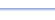Program: PERCENT

PERCENT

• Calculates the percent that the first number is of the second number.

Useful for:

• SAT
• ACT
• Basic Math
• Algebra 1
• Geometry

See full list of programs

Example

Q: What percent is 17 of 25?

Solution:

Run PERCENT.  How?

Enter 17 for PART.
Enter 25 for WHOLE.

The program reports:

Interpretation: It is 68%.------------------->